085-1263404

### My Journal

All things Mathematical
25 Nov 2018
##### Question 1

Two pegs are fixed at points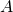and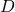in the same horizontal line. One end of a light string is attached toand the other end to. When masses of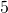and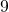lb. are attached to points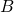and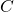, respectively, on the string, the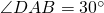and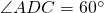. Find the tension, in lb. wt., in each of the segments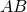,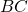,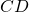of the string, correct in each case to one significant figure.

##### Question 2

Three particles weighing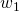,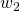,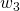are placed, respectively, at points the coordinates of which are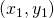,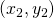,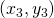. If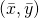are the coordinates of the centre of gravity of the system, show that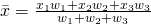,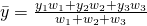Particles of weight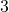,,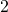and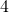grams are placed, respectively, at the points,,,, the coordinate of which are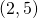,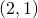,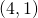,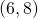respectively. Find the position of the centre of gravity of the system.

What additional weight must be placed atif the centre of gravity is required to be at the mid-point of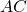?

##### Question 3

What is meant by the velocity of a particle relative to another particle?

Explain, with the aid of a diagram, how the velocity ofrelative tomay be found if the velocities ofandare known.

The velocity of a ship relative to a steady wind is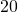m.p.h. in the direction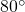North of East and the velocity of a boat relative to the same wind is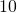m.p.h. in the direction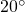South of West. Find the velocity (in magnitude and direction) of the ship relative to the  boat.

##### Question 4

A car weighing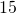cwt. is descending an incline ofin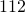. The speed of the car is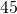m.p.h. and it is accelerating at the rate of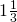ft. per sec.. If the frictional resistance to motion is equivalent tolb. wt., find the horse-power at which the car is working.

If the car travels in a straight line on a horizontal track against a frictional resistance to motion of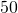lb. wt., find the greatest speed it attains if it developshorse-power.

##### Question 5

If three forces acting at a point are in equilibrium, prove that each force is proportional to the sine of the angles between the lines of action of the other two forces. The perpendiculars drawn from the vertices of an acute – angled triangle meet at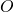. The perpendiculars fromto the sides of the triangles are the lines of action of three forces. Each force acts away fromand is proportional to the length of the side to which it is perpendicular. Prove that the three forces are in equilibrium.

##### Question 6

Derive an expression, in terms of the angle of projection and the initial velocity, for (i) the range, (ii) the greatest height reached by a projectile.

Two particles are projected from a pointwith the same initial velocity at angles of elevation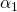and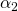. If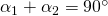, show that the range of each of the two particles is the same.

If the initial velocity of each of the particles if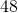ft. per second and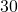ft. is the greatest height reached by one of the particles, find the greatest height reached by the other particle.

##### Question 7

Define simple harmonic motion.

A particle is moving in a striaght line with simple harmonic motion. When it iscm. from its mean position, its velocity and acceleration arecm. per sec. andcm. per sec., respectively. Find

(i) the greatest velocity of the particle,

(ii) the period of the motion,

(iii) the average velocity of the particle as it travels from rest to rest.

##### Question 8

A circular piece of tin rotates at the rate ofrevolutions per minute about an axis through its centre, the axis being perpendicular to the plane of the tin.andare two poitns on the tin such that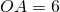inches,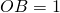ft.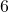ins. and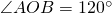. Weights oflb. andlb. are placed, respectively, atand. Find the magnitude and direction of the resultant centrifugal force on the axis.

Ifis a point on the tin such that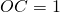ft.,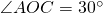and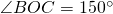, what weight must be placed atif the resultant centrifugal force is to act along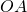?

##### Question 9

A vessel in the shape of a cube has internal edges eachft. long. A liquid fills the vessel to a height ofinches and a second liquid which does not mix the the first occupies the remainder of the vessel. On each vertical side the total thrust on that part in contact with one liquid is equal to the total thrust on that part in contact with the other. Show that the specific gravities of the two liquids are in the ratio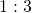.

##### Citation:

State Examinations Commission (2018). State Examination Commission. Accessed at: https://www.examinations.ie/?l=en&mc=au&sc=ru

Malone, D and Murray, H. (2016). Archive of Maths State Exams Papers. Accessed at: http://archive.maths.nuim.ie/staff/dmalone/StateExamPapers/

##### Licence:

“Contains Irish Public Sector Information licensed under a Creative Commons Attribution 4.0 International (CC BY 4.0) licence”.

The EU Directive 2003/98/EC on the re-use of public sector information, its amendment EU Directive 2013/37/EC, its transposed Irish Statutory Instruments S.I. No. 279/2005, S.I No. 103/2008, and S.I. No. 525/2015, and related Circulars issued by the Department of Finance (Circular 32/05), and Department of Public Expenditure and Reform (Circular 16/15 and Circular 12/16).

Note. Circular 12/2016: Licence for Re-Use of Public Sector Information adopts CC-BY as the standard PSI licence, and notes that the open standard licence identified in this Circular supersedes PSI General Licence No: 2005/08/01.